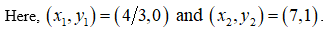# Find the equation of the line through the points (4/3,0) and (7,1)( Write your answer in the form y=mx+b.

Question
11 views

Find the equation of the line through the points (4/3,0) and (7,1)( Write your answer in the form y=mx+b.

check_circle

Step 1

Consider the given two points (4/3, 0) and (7, 1).

The general equation of the line is y = mx + b. If two points are given, then point slope formula is,

Step 2Step 3

The slope is calculat...

### Want to see the full answer?

See Solution

#### Want to see this answer and more?

Solutions are written by subject experts who are available 24/7. Questions are typically answered within 1 hour.*

See Solution
*Response times may vary by subject and question.
Tagged in

### Other